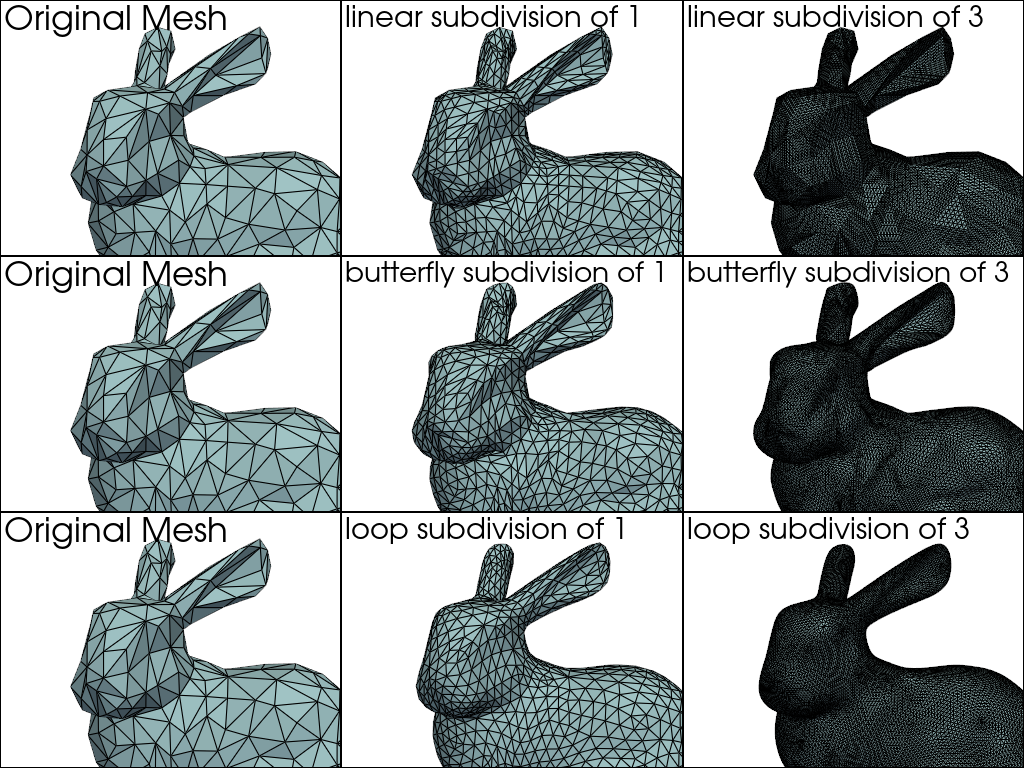# Subdivide Cells#

Increase the number of triangles in a single, connected triangular mesh.

The `pyvista.PolyDataFilters.subdivide()` filter utilizes three different subdivision algorithms to subdivide a mesh’s cells: butterfly, loop, or linear.

```import pyvista as pv
from pyvista import examples
```

First, let’s load a triangulated mesh to subdivide. We can use the `pyvista.DataSetFilters.triangulate()` filter to ensure the mesh we are using is purely triangles.

```mesh = examples.download_bunny_coarse().triangulate()

cpos = [
(-0.02788175062966399, 0.19293295656233056, 0.4334449972621349),
(-0.053260899930287015, 0.08881197167521734, -9.016948161029588e-05),
(-0.10170607813337212, 0.9686438023715356, -0.22668272496584665),
]
```

Now, lets do a few subdivisions with the mesh and compare the results. Below is a helper function to make a comparison plot of thee different subdivisions.

```def plot_subdivisions(mesh, a, b):
display_args = dict(show_edges=True, color=True)
p = pv.Plotter(shape=(3, 3))

for i in range(3):
p.subplot(i, 0)

def row_plot(row, subfilter):
subs = [a, b]
for i in range(2):
p.subplot(row, i + 1)
p.add_text(f"{subfilter} subdivision of {subs[i]}")

row_plot(0, "linear")
row_plot(1, "butterfly")
row_plot(2, "loop")

p.view_isometric()
return p
```

Run the subdivisions for 1 and 3 levels.

```plotter = plot_subdivisions(mesh, 1, 3)
plotter.camera_position = cpos
plotter.show()
```Total running time of the script: ( 0 minutes 0.232 seconds)

Gallery generated by Sphinx-Gallery